## Section 2 - The Basics

### 2.1 Drawing scales and using units for RealType

In this example, we draw scales and both x- and y-axis. The example P2_01 is almost the same as the previous example. This time we define our RealTypes time and height with units:
`time = new RealType("time", SI.second, null);`
and
`height = new RealType("height", SI.meter, null);`

The first argument in the constructor is the name (a Java String) of the RealType. This name will by used to label the axes. You can get the name of a RealType with the method RealType.getName(). The method RealType.getRealTypebyName( String name ) will return the RealType whose name is "name". Note that two RealTypes are equal if their names are equal.
The second argument is the unit of the RealType. VisAD defines all SI units (ampere, candela, kelvin, kilogram, meter, second, mole and radian) and provides methods for defining your own units. In section 2.7 we will create a new unit. By the way, you can get a RealType's unit with the method RealType.getDefaultUnit().
The third argument in the constructor is the default set of the RealType. We shall ignore the set for the time being.

The next addition we make to the first example is the call

`GraphicsModeControl dispGMC = (GraphicsModeControl) display.getGraphicsModeControl();`
that defines the variable dispGMC as display's GraphicsModeControl, and the subsequent call
`dispGMC.setScaleEnable(true);`
which specifies that scales should be drawn.

Running program P2_01 generates a window like the screen shot below.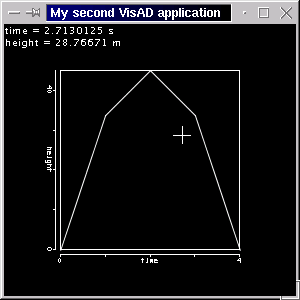Note that the axes are now labelled, and the cursor's position (time and height) is correctly given in seconds and meters.

[Top] [Home] [Back]

### 2.2 Scaling axes

You may have noticed that both axes were automatically scaled. In this section we will show how to manually scale the axes. Before we do that, we'll make another change in our program. We shall now use a different Set for the RealType time. In the previous examples, time Data was given by an Integer1DSet, the time_set. Now time_set will be a Linear1DSet. Note the arguments that this Set takes.
`time_set = new Linear1DSet(time, -3.0, 3.0, 5);`
We still use the same 5 height values, but now the parabola is correctly placed in the graph, that means time doesn't range from 0 to 4, because we use an adequate Set. Note that the parabola is given by height = 45 - 5 * time^2. The Integer1DSet was used initially because we were not interested in the mathematical correctness, but only in having a set of 5 values.

After adding the heightMap to the display, we scale the y-axis (remember, heightMap has YAxis as DisplayRealType) with

`heightMap.setRange( 0.0, 50.0);`
The following figure is a screen shot of the example P2_02. Note that the y-axis is now scaled from 0 to 50.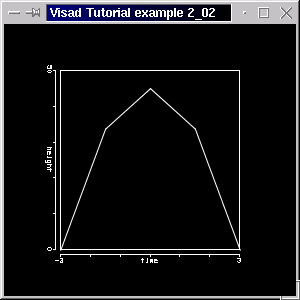[Top] [Home] [Back]

### 2.3 Plotting points by using a different MathType

We will now use a different MathType to organize our data in a different way, and see how the data structure gets depicted in a coherent way.
Note that our previous MathType indicates a continuous function. Our new MathType, organized as
`( index -> (time, height) )`
suggests, on the other hand, a set of (time, height) points, which are indexed by an Integer1DSet (index_set). The difference is not a trivial one. A continuous line like that of the previous example might represent the theoretical values of a continuous function and therefore is plotted as such. The latter MathType might represent a set of values from an experiment which should, therefore, be plotted disconnected.
As said, we are going to use an Integer1DSet for index.  In order to organize time and height, we will use a Tuple:
```private RealTupleType t_h_tuple;
t_h_tuple = new RealTupleType( time, height);```
The FunctionType now becomes:
`func_i_tuple = new FunctionType( index, t_h_tuple);`
And the FlatField is changed to include this:
```vals_ff = new FlatField( func_i_tuple, index_set);
vals_ff.setSamples( point_vals );```
The x-axis and the y-axis will be arbitrarily rescaled in the range from -4 to 4 and -10 to 50, respectively, using setRange().

The code for the complete example 2_03 is as follows:

```
// Import needed classes

import java.rmi.RemoteException;
import java.awt.*;
import javax.swing.*;

/**
Data are organized as MathType ( index  -> ( time, height )  ) and
represent some points from the parabola of the previous example
Data are indexed (time, height) points and get depicted as such.
Run program with java P2_03
*/

public class P2_03{

// Declare variables
// The quantities to be displayed in x- and y-axes: time and height, respectively
// Our index is alos a RealType

private RealType time, height, index;

// A Tuple, to pack time and height together

private RealTupleType t_h_tuple;

// The function ( time(i), height(i) ), where i = index,
// represented by ( index -> ( time, height) )
// ( time, height) are a Tuple, so we have a FunctionType
// from index to a tuple

private FunctionType func_i_tuple;

// Our Data values, the points, are now indexed by the Set

private Set index_set;

// The Data class FlatField, which will hold time and height data.
// time data are implicitely given by the Set time_set

private FlatField vals_ff;

// The DataReference from the data to display

private DataReferenceImpl data_ref;

// The 2D display, and its the maps

private DisplayImpl display;
private ScalarMap timeMap, heightMap;

public P2_03 (String []args)

// Create the quantities
// x and y are measured in SI meters
// Use RealType(String name, Unit u,  Set set), set is null

time = new RealType("time", SI.second, null);
height = new RealType("height", SI.meter, null);

// Organize time and height in a Tuple

t_h_tuple = new RealTupleType( time, height);

// Index has no unit, just a name

index = new RealType("index");

// Create a FunctionType ( index -> ( time, height) )
// Use FunctionType(MathType domain, MathType range)

func_i_tuple = new FunctionType( index, t_h_tuple);

// Create the x_set, with 5 values, but this time using a
// Integer1DSet(MathType type, int length)

index_set = new Integer1DSet(index, 5);

// These are our actual data values for time and height
// Note that these values correspond to the parabola of the
// previous examples. The y (height) values are the same, but the x (time)
// are now given given.

float[][] point_vals = new float[][]{{-3.0f, -1.5f, 0.0f, 1.5f, 3.0f,},
{0.0f, 33.75f, 45.0f, 33.75f, 0.0f,} };

// Create a FlatField, that is the Data class for the samples
// Use FlatField(FunctionType type, Set domain_set)

vals_ff = new FlatField( func_i_tuple, index_set);

// and put the height values above in it

vals_ff.setSamples( point_vals );

// Create Display and its maps

// A 2D display

display = new DisplayImplJ2D("display1");

// Get display's graphic mode control and draw scales

GraphicsModeControl dispGMC = (GraphicsModeControl) display.getGraphicsModeControl();
dispGMC.setScaleEnable(true);

// Create the ScalarMaps: quantity time is to be displayed along XAxis
// and height along YAxis
// Use ScalarMap(ScalarType scalar, DisplayRealType display_scalar)

timeMap = new ScalarMap( time, Display.XAxis );
heightMap = new ScalarMap( height, Display.YAxis );

// Scale heightMap. This will scale the y-axis, because heightMap has DisplayRealType YAXIS
// We simply choose the range from -4 to 4 for the x-axis
// and -10.0 to 50.0 for

timeMap.setRange( -4.0, 4.0);
heightMap.setRange( -10.0, 50.0);

// Create a data reference and set the FlatField as our data

data_ref = new DataReferenceImpl("data_ref");

data_ref.setData( vals_ff );

// Create application window, put display into it

JFrame jframe = new JFrame("VisAD Tutorial example 2_03");

// Set window size and make it visible

jframe.setSize(300, 300);
jframe.setVisible(true);

}

public static void main(String[] args)
{
new P2_03(args);
}

}
```

The source code is available here. If you compile it and run with java P2_03 you should be able to see the following window.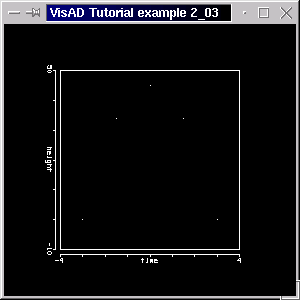As expected, our data consisting of a set of points was plotted as such (on some high-resolution screens the points may be so small - a single pixel - that they are nearly invisible).

Note that if you call

```dispGMC.setPointMode(true);
```
where dispGMC is the GraphicsModeControl you can draw all lines in a display as points, without changing the MathType. The advantage is that you don't need to change the MathType, but the disadvantage is that you will only be able to draw your data as points.

[Top] [Home] [Back]

### 2.4 Using a ConstantMap to Change Data Depiction Attributes

In the above figure, our data were plotted as the disconnected points. On some displays the points are so small that they are difficult to see, so you might want them larger or you simply might want them plotted with some color. We will now change some basic attributes of those points by using color and points ConstantMaps.  This is done by defining ConstantMaps like

```ConstantMap[] pointsCMap = { new ConstantMap( 1.0f, Display.Red ),
new ConstantMap( 0.0f, Display.Green ),
new ConstantMap( 0.0f, Display.Blue ),
new ConstantMap( 3.50f, Display.PointSize )  };
```
Note that Display.Red is at its maximum (1.0f) and Display.PointSize defines the size of the points in pixels. The change is implemented by linking this ConstantMap[] with the display using the call:
`display.addReference( data_ref, pointsCMap );`

The source code is available here. If you compile it and run with java P2_04 you should be able to see the following window.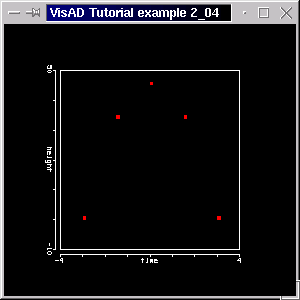The points are now displayed as red color-filled squares, 3 pixels on a side.

[Top] [Home] [Back]

### 2.5 Using a SelectRange Map to limit plotting and adding two DataReferences to a display

We now combine examples 2_02 and 2_04 above to make a display that shows data plotted as both single points and as a line. To do this we will use one display object, but we will require two FunctionTypes, two FlatFields, and two DataReferences.
First, create a Linear1DSet to define the sampling.
```int LENGTH = 25;
time_set = new Linear1DSet(time, -3.0, 3.0, LENGTH );```
Next, in addition to func_i_tuple created in the previous example, we need to create:
`func_time_height = new FunctionType(time, height);`
Now, we need to create two arrays to hold our data values, and fill them appropriately:
`float[][] d_vals = time_set.getSamples( true);`
`float[][] h_vals = new float[LENGTH];`
We generate values for the line with a for-loop.
```for(int i = 0; i < LENGTH; i++)
h_vals[i] =  45.0f - 5.0f * (float) (d_vals[i]*d_vals[i]);

```
This provides the data to make a curve of 25 connected points.

Another new feature in this example is the use of a ScalarMap with a SelectRange as DisplayRealType to select the time range to be displayed. In section 2.2 we scaled the y-axis. If we had scaled it so that our data would be outside the display box, data would still have been displayed (but outside the box!). A ScalarMap with SelectRange as DisplayRealType will trim data depiction, so that only the data inside the valid range will be drawn. (You might want to combine a ScalarMap.setRange() call with a ScalarMap with SelectRange as DisplayRealType, as the former allows you to choose the range of the depicted RealType (thus scaling an axis) and the latter allows to choose the range in which the RealType can be draw (values ouside the range will be cut). You can also see section 2.11, where the difference between them should become clear.)
We create such a ScalarMap with

`timeRangeMap = new ScalarMap( time, Display.SelectRange );`
add it to a display like we would do with any other ScalarMap:
`display.addMap( timeRangeMap );`
Then we get this ScalarMap's RangeControl
`RangeControl timeRangeControl = (RangeControl) timeRangeMap.getControl();`
To make the data displayed or visible in the range from -2 to 4, define the range
`float[] timeRange = { -2.0f, 4.0f };`
and finally implement the changes by calling
`timeRangeControl.setRange( timeRange );`
The complete source code for this example is available here. If you compile it and run with java P2_05 you should be able to see the following window.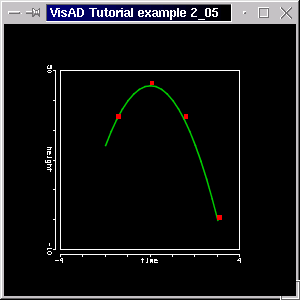Note that data outside the range from -2 to 4 is not shown. (Compare with the previous figures, in which both line and points existed in the range -3 to 3.) Note too the smoother curve, indicating the use of more data (for the line). Of course, the plots of the point value data and the data describing the line are completely independent (try changing their values in the code).

[Top] [Home] [Back]

### 2.6 Extending the MathType and using Display.RGB

In the previous examples, our line had the following MathType
`( time -> height )`
We mapped time to XAxis and height to YAxis. We will now extend this MathType to
`( time -> (height, speed) )`
where height and speed are RealTypes and form a Tuple:
`h_s_tuple = new RealTupleType(height, speed);`
Note that speed is the first derivative of height with respect to time. (You might like to know that the interface Function (subinterface of Data) actually includes a method to calculate the derivative of a Function with respect to a RealType. Remember that in VisAD a FlatField represents a mathematical function. It overrides the Data.derivative() method, with which you can calculate the derivative of a function, that is of a FlatField, with respect to a quantity, that is a RealType. But in this example we shall calculate the derivative in a for-loop. See section 4_07 for how to use Data.derivative().)
Our FunctionType is now a function
`func_t_tuple = new FunctionType(time, h_s_tuple);`
Note that the array which will hold the height and speed values is now dimensioned like float[ number_of_range_components ][ number_of_range_samples], that is float[LENGTH]:
```float[][] h_s_vals = new float[LENGTH];
```

We compute the values for height and speed with for-loop:
```for(int i = 0; i < LENGTH; i++){

// height values...
h_s_vals[i] =  45.0f - 5.0f * (float) (t_vals[i]*t_vals[i]);

// ...and speed values: the derivative of the above function
h_s_vals[i] =  - 10.0f * (float) t_vals[i];
}

```

We create the ScalarMap

`speedRGBMap = new ScalarMap( speed, Display.RGB );`
to display speed in RGB color and then we add this ScalarMap to the display. The source code for this example is available here. The figure below is a screen shot of this program.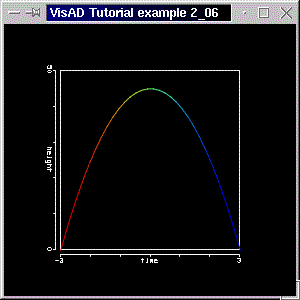Note that speed is signed, so positive (upward) values are colored red and negative (downward) values are colored blue. The color look-up table ranges from blue, through green to red. The color table is automatically adjusted to the RealType it's attached to, so that the minimum value of the RealType is mapped to the minimum value of the color table (blue) and the maximum value of the RealType corresponds to that of the color table (red). Intermediate values are linearly interpolated.
In section 3 we will learn how to color a RealType using other DisplayRealTypes (like Red, Green and Blue, rather than RGB, which is attached to a pseudo color look-up table). In section 4 we will define and use a new color table.

### [Top] [Home] [Back] 2.7 New Units, and changing line width with GraphicsModeControl

This example is almost the same as the previous example. This first little change regards the units of speed. We declare our new Unit with
`Unit mps;`
define it with
`mps = SI.meter.divide( SI.second );`
and refine speed with the new unit:
`speed = new RealType("speed", mps, null);`
That is, our new Unit is represented by mps and is simply SI.meter divided by SI.second, or simply meters per second. We define speed's units as mps. We also invert the ScalarMaps and map height to RGB and speed to YAxis, as shown below:
`speedYMap = new ScalarMap( speed, Display.YAxis );`
and
`heightRGBMap = new ScalarMap( height, Display.RGB );`
Just another little change is the call
`dispGMC.setLineWidth( 3.0f );`
which results in thicker lines for the display. The source code for this example is available here and below is a screen shot of the program.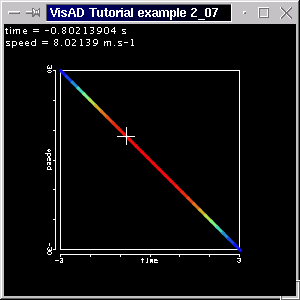Note that speed is displayed in the y-axis, and the values for time and speed at the cursor's location are correctly given in the proper units. Remember, not speed but height is mapped to color, so now we see red near time = zero, where the height is at the maximum, and blue at both ends where heights are near the minimum.

You might want to know, that the method GraphicsModeControl.setPointWidth( float size ) changes the point size of a display (but is over-ridden by ConstantMaps to Display.PointSize, as used in section 2.4). Although the GraphicsModeControl provides you extensive controls over data depiction, VisAD provides a user interface for the it. In order to make full use of this interface, we shall only introduce it in section 3.8.

[Top] [Home] [Back]

### 2.8 Plotting two quantities on same axis

In this section we restructure the MathType of the previous program
`( time -> (height, speed) )`
as
`( time -> height )`
and
`( time -> speed )`
We will need a FunctionType for each of the above functions
`func_t_h = new FunctionType( time, height );`
and
`func_t_s = new FunctionType( time, speed );`
as well as FlatFields and DataReferences. The RealTypes height and speed are both mapped to y-axis, and speed's DataReference include a yellow ConstantMap, to distinguish speed from height. The speed's axis is equally colored yellow, but with the call ScalarMap.setScaleColor( float[] speedColor ). The complete code is available here. The program generates a window like the figure below: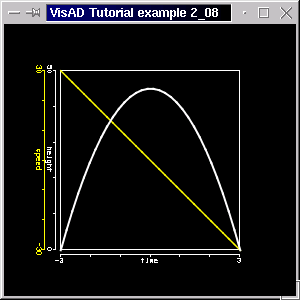You might also want to know that is possible to prevent an extra axis to be drawn, even though you might have added a corresponding ScalarMap (with XAxis, YAxis or ZAxis) to the display. This is achieved by calling

`ScalarMap.setScaleEnable( false );`
The code for this example already includes such a call. Just uncomment the line with speedYMap.setScaleEnable(false) to prevent the speed axis from being drawn.

[Top] [Home] [Back]

### 2.9 Using a Gridded1DSet

So far we have used Integer1DSet and Linear1DSet, as our time (x-axis) domain. Both sets are finite arithmetic progression of values. Your data might not be in such a progression or you might, for whatever reason, want to use some values which are more densely sampled in some region as it is in others. Our next example considers such a case.

We have a Gaussian distribution curve, but we are more concerned with the region around the maximum. We have our points concentrated around this region, and only a few samples at the base of the curve. Our Gridded1DSet is defined as follows

`x_set = new Gridded1DSet(x, x_vals, LENGTH);`
where x is the quantity to be displayed on the x-axis, x_vals is an array float[LENGTH] with the x values and LENGTH is simply the number of values. The corresponding values for y are given further down in the program. We also have a ScalarMap with RealType y mapped to Display.RGB added to our display. This will color the curve according to y. The source code for this example is available here. The figure below is a screen shot of this program.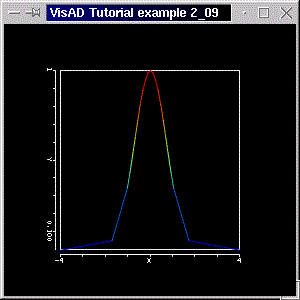Please note the varying sampling distances, indicating the use of the Gridded1DSet.

[Top] [Home] [Back]

### 2.10 Using a RangeWidget

In this section we introduce the second VisAD User Interface (the first VisAD User Interface is a display!). We saw in section 2.2 how we can scale axes by calling ScalarMap.setRange( double low, double hi ). Although this method may suffice, in some cases you will want to be able to interactively change an axis range. To do this VisAD provides a user interface, the RangeWidget. We shall use example program P2_05 and add a RangeWidget to it.

To create a RangeWidget we simply declare one:

```private RangeWidget ranWid;
```

Note that we have added the import statement

```import visad.util.*;
```
In the directory visad/util you will find other useful user interfaces.

We actually create such a widget with

```ranWid = new RangeWidget( timeMap );
```
Remeber, timeMap is the ScalarMap which of the RealType time and it's mapped to the x-axis. The final step it to add the widget to the window. You can see the code here. Running the program with "java tutorial.s2.P2_10" (and typing in the same range values given below) you should get a window like the screen shot below.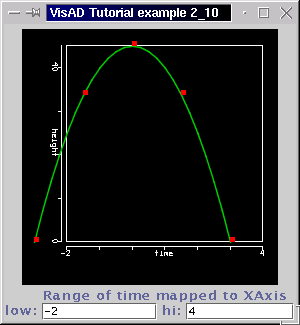Note the range of the RealType time is between -2 and 4 (the curve only goes to 3 because our data, that is the domain set, is only defined between -3 and 3). By typing in a value in the text field and then pressing the "enter" key the display gets redrawn with the new range. You should run the example and try the widget out!

You should try to create a RangeWidget for the y-axis. You'd only need to create a widget for the heightMap and then add it to window.

[Top] [Home] [Back]

### 2.11 Using a SelectRangeWidget

In this section we introduce the SelectRangeWidget, which allows you to select the range in which data will be drawn. In section 2.5 we used created a ScalarMap
```timeRangeMap = new ScalarMap( time, Display.SelectRange );
```
added it to the display, then we got its RangeControl
`RangeControl timeRangeControl = (RangeControl) timeRangeMap.getControl();`
defined a range
`float[] timeRange = { -2.0f, 4.0f };`
and finally implemented the changes by calling
`timeRangeControl.setRange( timeRange );`
This is fine in case that the selected range doesn't (or shouldn't) change during runtime. To interactively change Display.SelectRange bounds, VisAD provides the SelectRangeWidget. We shall use example program P2_10 to show this widget. In the program, we declare a SelectRangeWidget
```SelectRangeWidget selRanWid;
```
We also need a map like
```timeRangeMap = new ScalarMap( time, Display.SelectRange );
```
which determines that the RealType time will have a range which can be selected, as done in section 2.5. To create the widget we call
```selRanWid = new SelectRangeWidget( timeRangeMap );
```
We then add this widget to the window as we have done with the previous widget. You can see the complete code here. Running the program with "java tutorial.s2.P2_11" you should get a window with a display and with the two widgets. See the screenshot below.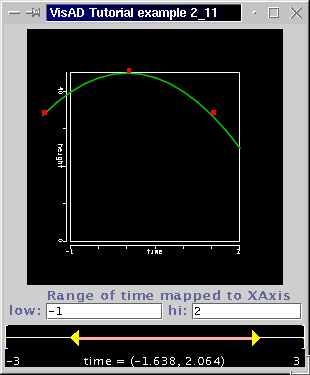Click and drag one of the yellow triangles to change one of the boundaries. Click and drag in the middle of the range to move both ends.

If you are confused with the two widgets, then we urge you to run the example and try them out. The RangeWidget is responsible for "scaling the axis", whereas the SelectRangeWidget is responsible for selecting the range in which data can be drawn. We said in section 2.5 that you might want to combine both together in an application. Indeed, if by scaling the axis data gets drawn outside the display, you might use the SelectRangeWidget to avoid that.

In the next section we make use of 2D Sets, and introduce images.

[Top] [Home] [Back]